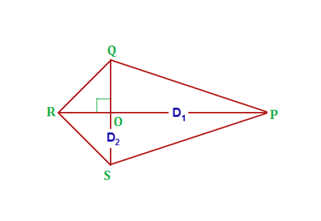# Area of a Kite Formula

A quadrilateral is a polygon which has 4 vertices and 4 sides enclosing 4 angles. Kite is a special quadrilateral in which each pair of the consecutive sides is congruent, but the opposite sides are not congruent. Rhombus is a kite with all the four sides congruent.Properties of a Kite:

• Angles between unequal sides are equal.
• A kite can be viewed as a pair of congruent triangles with a common base.
• Diagonals of a kite intersect each other at right angles.
• The longer diagonal is the perpendicular bisector of the shorter diagonal.
• A kite is symmetrical about its main diagonal.
• The shorter diagonal divides the kite into two isosceles triangles.

## Formula for Area of a Quadrilateral

The diagonals of a kite are perpendicular. Area of a kite is given as half of the product of the diagonals which is same as that of a rhombus. Area of a kite can be expressed by the formula:

• Area of Kite =
$$\begin{array}{l}\frac{1}{2}D_{1}D_{2}\end{array}$$

D1 = long diagonal of kite

D= short diagonal of kite

### Derivation for Area of a Kite:

Consider the area of the following kite PQRS.

Here the diagonals are PR and QS

Let diagonal PR =a and diagonal QS = b

Diagonals of a kite cut one another at right angles as shown by diagonal PR bisecting diagonal QS.

OQ = OS =

$$\begin{array}{l} \frac{OS}{2}=\frac{b}{2}\end{array}$$

Area of the kite = Area of triangle PQR + Area of triangle PSR

Area of Triangle =

$$\begin{array}{l}\frac{1}{2}\;base \times height\end{array}$$

Here, base = a and height = OQ = OS= b/2

Area of triangle PQR =

$$\begin{array}{l}\frac{1}{2}\times a\times \frac{b}{2}\end{array}$$

Area of triangle PSR=

$$\begin{array}{l}\frac{1}{2}\times a\times \frac{b}{2}\end{array}$$

Area of the kite =

$$\begin{array}{l}\frac{1}{2}\times a\times \frac{b}{2}\end{array}$$
+
$$\begin{array}{l}\frac{1}{2}\times a\times \frac{b}{2}\end{array}$$

=

$$\begin{array}{l}\frac{ab}{4}+\frac{ab}{4}\end{array}$$

=

$$\begin{array}{l}\frac{2ab}{4}=\frac{1}{2}ab\end{array}$$

Hence,

Area of the kite =

$$\begin{array}{l}\frac{1}{2}PR*QS\end{array}$$
= Half of the product of the diagonals

Note:

• If lengths of unequal sides are given, using Pythagoras theorem, the length of diagonals can be found. The area of a kite can be calculated by using the lengths of its diagonals.

### Solved Examples:

Example 1: Find the area of kite whose long and short diagonals are 22 cm and 12cm respectively.

Solution: Given,

Length of longer diagonal, D1= 22 cm

Length of shorter diagonal, D2= 12 cm

Area of Kite =

$$\begin{array}{l}\frac{1}{2}D_{1}D_{2}\end{array}$$

Area of kite =

$$\begin{array}{l}\frac{1}{2}\end{array}$$
x 22 x 12 = 132
$$\begin{array}{l}cm^{2}\end{array}$$

Example 2: Area of a kite is 126 cm² and one of its diagonal is 21cm long. Find the length of the other diagonal.

Solution: Given,

Area of a kite =126 cm²

Length of one diagonal = 21 cm

Area of Kite =

$$\begin{array}{l}\frac{1}{2}D_{1}D_{2}\end{array}$$

$$\begin{array}{l}126 = \frac{1}{2}\times 21\times D_{2}\end{array}$$

D2 = 12cm

To solve more problems on the topic and for video lessons on kite and other quadrilaterals, download BYJU’S – The Learning App.